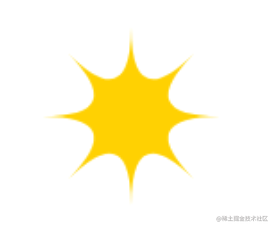# 在画布上绘图[入门canvas教程]

## svg

`````` <svg xmlns="http://www.w3.org/2000/svg">
<circle r="50" cx="50" cy="50" fill="red"></circle>
</svg>

xmlns属性将元素更改为其他XML命名空间。这个命名空间由URL标识, 指定了我们当前正在用的方言。HTML中不存在的 `<circle>``<rect>`标签在SVG中才有意义。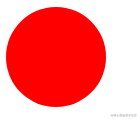``````let circle = document.querySelector('circle')
circle.setAttribute('fill', 'purple')

## 画布元素

``````<canvas width="100" height="100" />
let canvas = document.querySelector('canvas')
let context = canvas.getContext('2d');
context.fillStyle = "red";
context.fillRect(50, 50, 50, 50);

## 线和面

fillStyle用于控制形状填充的方式。 strokeStyle用于决定描边的样式。

``````let canvas = document.querySelector('canvas')
let context = canvas.getContext('2d');
context.strokeStyle="red";
context.strokeRect(5, 5, 50, 50);
context.lineWidth = 10
context.strokeRect(100, 5, 50, 50);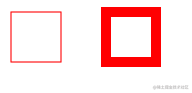## 路径

``````let canvas = document.querySelector('canvas')
let ctx = canvas.getContext('2d');
ctx.beginPath();
for (let y = 0; y < 100; y += 10) {
ctx.moveTo(10, y);
ctx.lineTo(90, y);
}

ctx.stroke()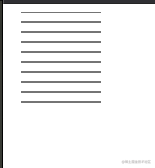``````let canvas = document.querySelector('canvas')
let ctx = canvas.getContext('2d');
ctx.beginPath();
ctx.moveTo(50, 10);
ctx.lineTo(10, 70);
ctx.lineTo(90, 70);
ctx.closePath();
ctx.fill()## 曲线

`quadraticCurveTo` 方法将曲线绘制到定点。为了确定线的曲率, 要给此方法提供 `控制点``目标点`.

``````let ctx = document.querySelector('canvas').getContext('2d');
ctx.beginPath();
ctx.moveTo(10, 90);
ctx.quadraticCurveTo(60, 10, 90, 90);
ctx.lineTo(60, 10);
ctx.closePath();
ctx.stroke();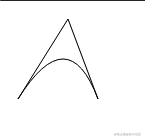bezierCurveTo 方法绘制了类似的曲线。这个曲线对于每个线路的断点都有两个控制点, 而不是单个控制点。

``````let ctx = document.querySelector('canvas').getContext('2d');
ctx.beginPath();
ctx.moveTo(10, 90);
// 控制点1(10, 10) 控制点2(90, 10) 目标点(50, 50)
ctx.bezierCurveTo(10, 10, 90, 10, 50, 90)
ctx.lineTo(90, 10)
ctx.lineTo(10, 10)
ctx.closePath()
ctx.stroke()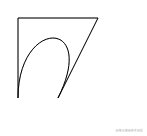arc方法是一种绘制会沿着圆的边缘弯曲的线的方法。它需要一对弧的中心坐标、一个半径、以及起始角和终止角。

``````let ctx = document.querySelector('canvas').getContext('2d');
ctx.beginPath();
ctx.arc(50, 50, 50, 0, 7);
ctx.arc(150, 50, 50, 0, 0.5 * Math.PI);
ctx.stroke()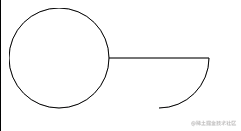## 绘制饼图

``````const result = [
{ name: 'A', count: 100, color: 'red' },
{ name: 'B', count: 50, color: 'blue' },
{ name: 'C', count: 80, color: 'pink' }
]

``````const results = [
{ name: 'B', count: 50, color: 'blue' },
{ name: 'A', count: 100, color: 'red' },
{ name: 'C', count: 80, color: 'pink' }
]

let ctx = document.querySelector('canvas').getContext('2d');
let total = results.reduce((sum, { count }) => sum + count ,0);
let currentAngle = 0;

for (let result of results) {
let sliceAngle = (result.count / total) * 2 * Math.PI;
ctx.beginPath();
ctx.arc(100, 100, 100, currentAngle, currentAngle + sliceAngle);
currentAngle +=sliceAngle;
ctx.lineTo(100, 100);
ctx.fillStyle = result.color;
ctx.fill()
}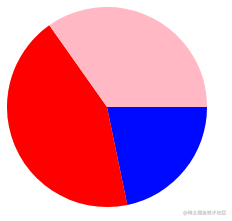## 添加文本

2D画布绘图上下文提供了 `fillText``strokeText`方法。后者可用于勾画字母轮廓, 但是通常filleText一般是我们经常用到的。

``````let ctx = document.querySelector('canvas').getContext('2d')
ctx.font = "30px Georgia"
ctx.fillStyle = "red"
ctx.fillText('i am summer', 10, 50);

``````ctx.font = "italic 30px Georgia"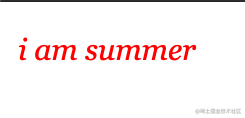fillText 和 strokeText 的最后两个参数提供了绘制字体的位置。默认情况下, 它们指示文本的字母基线开始的位置, 即字母所“站”的线, 不计算j或者p等字母的悬挂部分。我们可以通过将 `textAlign` 属性设置为 “end” 或者 “center”来更改水平位置, 或者通过将 `textBaseline` 设置为 "top" "middle" 来更改垂直位置。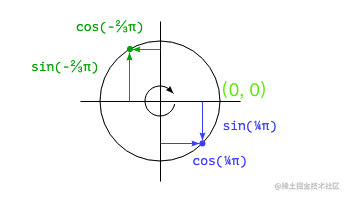`Math.cos``Math.sin` 可以用于计算原点为(0, 0), 半径为1的圆周上的点。这两个函数都将它们的参数解释为这个圆上的位置, 零表示圆最右侧的点. 顺时针方向运动到2π将会绕过整个圆周. Math.cos可以计算出对应点的x坐标, Math.sin可以计算出对应点的y坐标。

``````const results = [
{ name: 'B', count: 50, color: 'blue' },
{ name: 'A', count: 50, color: 'red' },
{ name: 'C', count: 50, color: 'pink' },
{ name: 'D', count: 50, color: 'yellow' }
]

let cx = document.querySelector("canvas").getContext("2d");
let total = results.reduce(function(sum, choice) {
return sum + choice.count;
}, 0);

let currentAngle = -0.5 * Math.PI;
let centerX = 300, centerY = 150;

results.forEach(function(result) {
let sliceAngle = (result.count / total) * 2 * Math.PI;
cx.beginPath();
cx.arc(centerX, centerY, 100, currentAngle, currentAngle + sliceAngle);
let middleAngle = currentAngle + 0.5 * sliceAngle;
let textX = Math.cos(middleAngle) * 120 + centerX;
let textY = Math.sin(middleAngle) * 120 + centerY;
cx.textBaseLine = "middle";
if (Math.cos(middleAngle) > 0) {
cx.textAlign = "left";
} else {
cx.textAlign = "right";
}
cx.font = "15px sans-serif";
cx.fillStyle = "black";
cx.fillText(result.name, textX, textY);

currentAngle += sliceAngle;
cx.lineTo(centerX, centerY);
cx.fillStyle = result.color;
cx.fill();
});

## 总结

• 菱形
`````` let ctx = document.querySelector("canvas").getContext("2d");
ctx.translate(100, 100)
ctx.rotate(Math.PI / 4);
ctx.fillStyle = "red";
ctx.fillRect(20, 20, 60, 60);
ctx.resetTransform();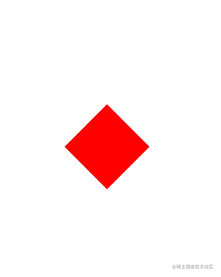• 弹簧
``````const x = 240, y =20;
let ctx = document.querySelector("canvas").getContext("2d");
ctx.beginPath();
ctx.moveTo(x, y);
for (let i = 0; i < 8; i++) {
ctx.lineTo(x + 80, y + i * 8 + 4);
ctx.lineTo(x, y + i * 8 + 8);
}
ctx.stroke();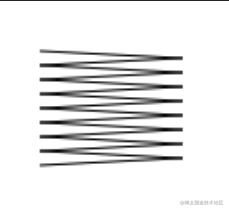• 旋涡
``````const x = 240, y =20;
let ctx = document.querySelector("canvas").getContext("2d");
let radius = 50, xCenter = x + radius, yCenter = y + radius;
ctx.beginPath();
ctx.moveTo(xCenter, yCenter);
for (let i = 0; i < 300; i++) {
let angle = i * Math.PI / 30;
let dist = radius * i / 300;
ctx.lineTo(xCenter + Math.cos(angle) * dist,
yCenter + Math.sin(angle) * dist);
}
ctx.stroke();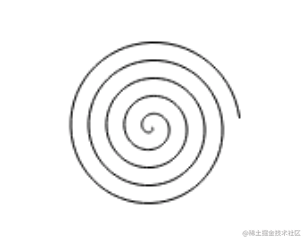• 黄色星星
``````const x = 240, y =20;
let ctx = document.querySelector("canvas").getContext("2d");
let radius = 50, xCenter = x + radius, yCenter = y + radius;
ctx.beginPath();
ctx.moveTo(xCenter + radius, yCenter);
for (let i = 1; i <= 8; i++) {
let angle = i * Math.PI / 4;
ctx.quadraticCurveTo(xCenter, yCenter,
xCenter + Math.cos(angle) * radius,
yCenter + Math.sin(angle) * radius);
}
ctx.fillStyle = "gold";
ctx.fill();